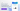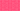Pricing# Profitability index formula and template

Written by

Before investing in any new project, it’s crucial to analyse its chances of profitability. This is where the profitability index is useful, giving an easily understandable ratio that can help with decision making.

## What is the profitability index rule?

The profitability index (PI) offers a way to measure the balance between an initial investment and its present value of future cash flows. It’s a simple ratio that indicates whether or not an investment project is likely to be profitable by showing the value created per investment unit. A general rule of thumb is that a PI greater than one indicates the project should go forward, while a PI below one indicates it will not be worth the investment.

The PI is also known as the value investment ratio (VIR) and profit investment ratio (PIR) in finance.

## What is the profitability index formula?

The profitability index formula shows the present value of future cash flows, divided by the initial project cost. It looks like this:

Profitability Index = Present Value of Future Cash Flows / Initial Investment

Another representation of the profitability index formula would be as follows:

Profitability Index = (Net Present Value + Initial Investment) / Initial Investment

After plugging numbers into the PI, you can decide whether to greenlight a given project. For example, imagine that you are planning a project that costs \$1000 in initial investment. Its anticipated return will be \$1,300. Plugging this into the equation, you can see:

Profitability Index = \$1,300 / \$1,000

Therefore, PI = 1.3.

Because the PI value is greater than one, the project will be profitable. With the profitability index, the higher the value, the more profitable the investment.

## Difference between NPV and profitability index

PI is very similar to the concept of net present value (NPV), but there are a few differences. Generally speaking, a positive NPV will correspond with a PI greater than one, while a negative NPV will track with a PI below one.

The main difference between NPV and profitability index is that the PI is represented as a ratio, so it won’t indicate the cash flow size. A profitability index number might be 1.5, but you wouldn’t necessarily know the capital expenditure required.

Here’s how you can calculate the NPV:

1. Identify all cash inflows and outflows.

2. Determine the appropriate discount rate.

3. Using the discount rate, find the present value of the cash inflows and outflows.

4. Add together all the present values.

The NPV shows you how profitable the project in question will be compared to alternative projects. A higher NPV indicates a higher rate of profit.

There are many advantages of profitability index rules:

• It is simple and easy to use.

• It helps rank projects in order of potential profitability.

• It takes the time value of money into consideration.

• It clearly indicates whether the investment will add positive or negative value.

By contrast, there are also a few limitations or disadvantages to the profitability index formula.

• It uses an estimate of the cost of capital.

• It may not be entirely accurate as it relies on estimations.

• It’s not 100% reliable when choosing between mutually exclusive projects.

## Profitability index template

You can use a profitability index template or table like the one below to plug in your values. A PI template helps give a visual representation of the present value of future cash flows in order to calculate your project’s PI.

 Initial Investment Required Discount Rate Year 1 2 3 4 5 Discount Factor Cash flow Present Value Discounted Value Present Value of Future Cash Flows Profitability Index

So, how do you calculate the profitability index? Simply enter your initial investment and discount rate, then use the profitability index formula to fill in the rest of the template.

## We can help

GoCardless helps you automate payment collection, cutting down on the amount of admin your team needs to deal with when chasing invoices. Find out how GoCardless can help you with ad hoc payments or recurring payments.